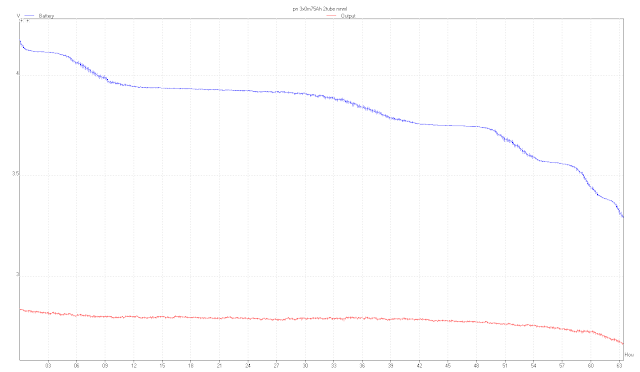## Thursday, 29 November 2018

### Observing a 170% conversion of battery energy into work

The basis of the Ringwood Energy Recycler circuit used in these tests is not particularly unusual - it's a simple blocking oscillator and it has a work-efficiency of approximately 85% (quantifiable-work performed for total energy supplied)

#### Fig. 1 - Circuit with current feedback to supply battery

Unquantified losses include resistive heat-loss in the switching transistor & diode, coil windings, and transistor biasing

The switching circuit operates at approx 67 kHz, in 2 half-cycles: a `Drain` step, followed by a `Recharge` step

#### Fig. 2 - Current paths in the system

The battery capacity can be characterised by discharging it using a resistor with a known value, over the terminal-voltage range 4.2V to 3.45V

Using a battery with 3 NiMH cells of 750mAh rated capacity, a fully charged battery supplied a 268 ohm (measured) resistor with an average power of 14.4mA x 3.86V for 42.5 hours, converting a total of 8504 Joules of energy

When the discharge resistor is replaced with the preliminary circuit (ie. the feedback diode is connected to a spare battery which is not the supply battery, but similar construction) the quantifiable work consists of two parts:-
- converting 3105 Joules to illuminate some LEDs
- recharging the spare battery by using 4238 Joules of energy which is being temporarily stored in the circuit as a by-product of the oscillator operation

The circuit provides this quantifiable work for a total of 30.5 hours, drawing an average of 20.1mA at 3.86V to draw a total of 8603 Joules (approximately matching the total energy, 8504 Joules, converted by the nominal 270 ohm resistor)

In this mode, the circuit draws the total energy available and converts this to 7343 Joules of quantifiable work, giving a quantifiable-work efficiency of 85%

When the feedback diode is re-connected to the supply mode (see Fig. 1), the circuit will operate for almost twice the duration of the conventional, non-feedback mode - the circuit now operates for a total of 60.5 hours, whilst drawing the same real average power, 20.1mA at 3.86V,  (compared to 30.5 hours duration for the non-feedback mode) with the loads remaining the same values

[The circuit can operate at the same power drain for longer because it is constantly re-charging its supply with a proportion of the energy which has been input - the actual supply current drained is pulsed, with an average of 20.1mA, whilst 10mA av. is recharged back into the battery, being interleaved each cycle similar to time-division-multiplexed operation (see Figs. 2 & 3), resulting in a 'virtual' current drain from the battery of (20.1mA - 10mA) = 10.1mA av.]

#### Fig. 3 -  Bipolar current pulses in supply connection (blue trace)

The total energy drawn by the circuit is now 16898 Joules

The efficiency of the oscillator circuit compared to its total energy drawn hasn't changed essentially:
the quantifiable work has increased to 14566 Joules, and these values give an efficiency of 14566 / 16898 = 86%

The overall system efficiency for quantifiable work from the original energy in the battery, however, is now 14566 / 8504 = 170%

The total system efficiency for conversion of energy in the whole system (battery + circuit) now becomes: 16898  / 8504 = 199%

The system is recycling its input energy by a factor of 1.99

The 'feedback to supply' mode has extended the duration for circuit operation and enabled the amount of useful energy available for the LEDs to be approximately doubled compared to the 'no feedback' arrangement

Although the total work converted by the switching circuit LEDs, on their own, remains less than the original supply of energy, and the energy converted by those circuit LEDs matches that of a passive resistor-driven LED arrangement (6159J vs. 6169J), the 'With-Feedback' current arrangement still enables the circuit to produce a level of light output slightly greater than the passive DC-drive arrangement (146 Ft-candles vs 140 approx.) but for approximately 34% longer duration (60.5 hours vs. 45 hours)

This resulting system behaviour provides a worthwhile gain, which has been enabled by the 200% conversion to system work (16898J) of the original store of energy in the battery (8504J)Fig. 4 - Blue trace: Battery discharge profile (No energy feedback); Red trace: LED drive level (DC volts)Fig. 5 - Blue trace: Battery discharge profile (With energy feedback); Red trace: LED drive level (DC volts)